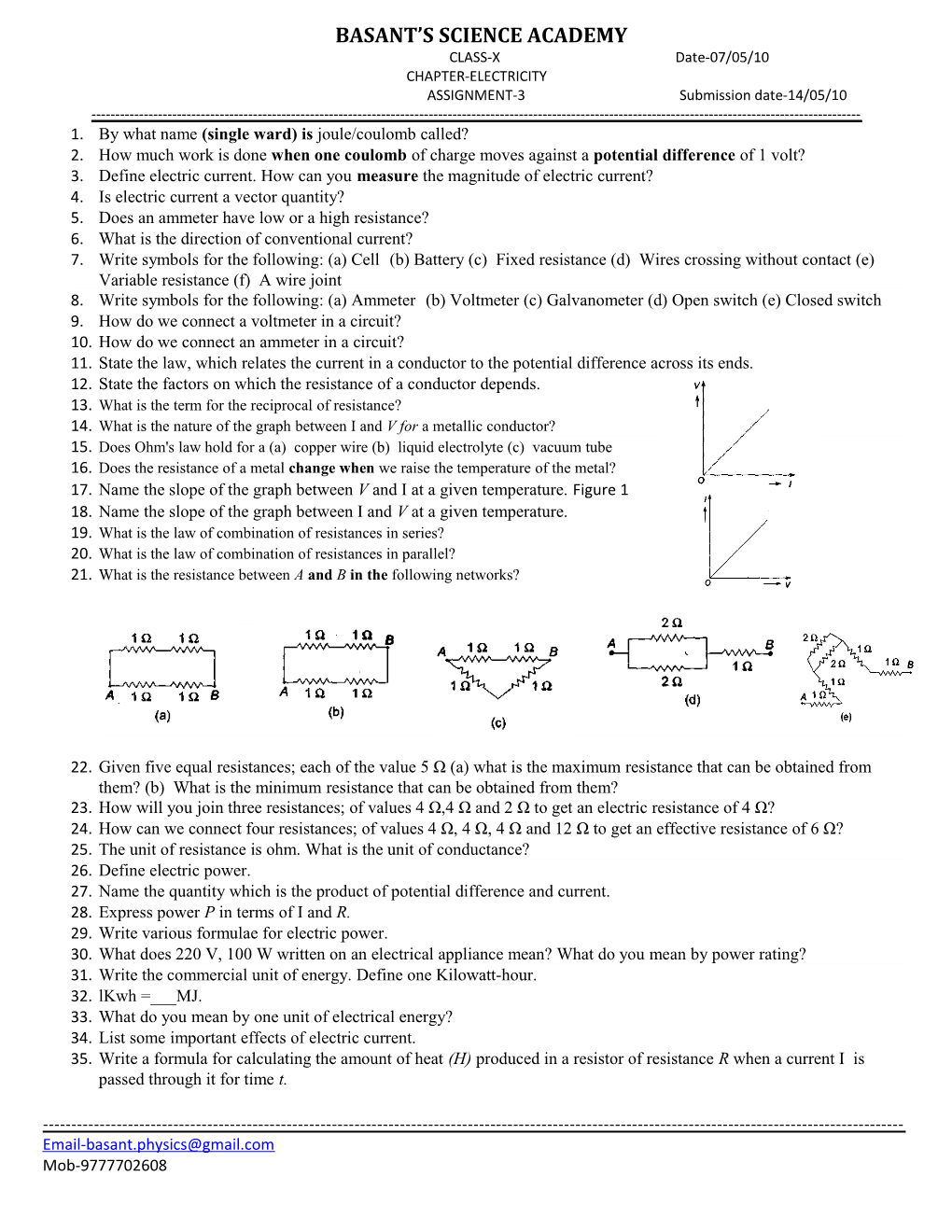CLASS-X Date-07/05/10

CHAPTER-ELECTRICITY

ASSIGNMENT-3 Submission date-14/05/10

------

1. By what name (single ward) isjoule/coulomb called?
2. How much work is done when one coulomb of charge moves against a potential difference of 1 volt?
3. Define electric current. How can you measure the magnitude of electric current?
4. Is electric current a vector quantity?
5. Does an ammeter have low or a high resistance?
6. What is the direction of conventional current?
7. Write symbols for the following: (a) Cell (b) Battery(c) Fixed resistance(d) Wires crossing without contact(e) Variable resistance(f) A wire joint
8. Write symbols for the following: (a) Ammeter (b) Voltmeter (c) Galvanometer (d) Open switch (e) Closed switch
9. How do we connect a voltmeter in a circuit?
10. How do we connect an ammeter in a circuit?
11. State the law, which relates the current in a conductor to the potential difference across its ends.
12. State the factors on which the resistance of a conductor depends.
13. What is the term for the reciprocal of resistance?
14. What is the nature of the graph between I and V for a metallic conductor?
15. Does Ohm's law hold for a(a) copper wire(b) liquid electrolyte(c) vacuum tube
16. Does the resistance of a metal change when we raise the temperature of the metal?
17. Name the slope of the graph between V and I at a given temperature.Figure 2
18. Name the slope of the graph between I and V at a given temperature.
19. What is the law of combination of resistances in series?
20. What is the law of combination of resistances in parallel?
21. What is the resistance between A and B in thefollowing networks?
1. Given five equal resistances; each of the value 5 Ω (a) what is the maximum resistance that can be obtained from them?(b) What is the minimum resistance that can be obtained from them?
2. How will you join three resistances; of values 4 Ω,4 Ω and 2 Ω to get an electric resistanceof 4 Ω?
3. How can we connect four resistances; of values 4 Ω, 4 Ω, 4 Ω and 12 Ω to get an effective resistance of 6 Ω?
4. The unit of resistance is ohm. What is the unit of conductance?
5. Define electric power.
6. Name the quantity which is the product of potential difference and current.
7. Express power P in terms of I and R.
8. Write various formulae for electric power.
9. What does 220 V, 100 W written on an electrical appliance mean? What do you mean by power rating?
10. Write the commercial unit of energy.Define one Kilowatt-hour.
11. lKwh =___MJ.
12. What do you mean by one unit of electrical energy?
13. List some important effects of electric current.
14. Write a formula for calculating the amount of heat (H) produced in a resistor of resistance R when a current I is passed through it for time t.

------

Mob-9777702608